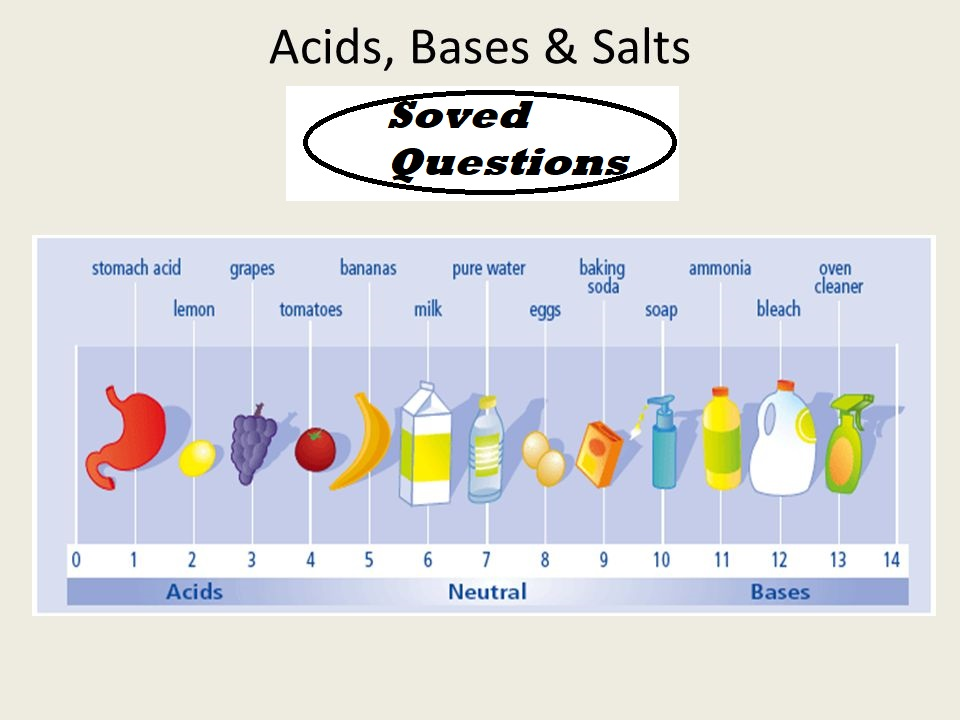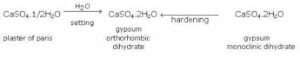# Acids Bases Salts Solved QuestionsAcids Bases Salts Solved Questions

All important questions are included in Acids Bases Salts Solved Questions.

Que1. Define the terms: Acid, alkali and salt.
Ans 1: An acid is a compound, which releases hydronium ions (H3O+) as the only positive ions in solution.
An alkali is a compound, which releases hydroxyl ions (OH) as the only negative ions in solution.
A salt is one of the products of neutralization between an acid and a base; water being the only other product.
OR
A salt gives positive ions other than H+ ion and negative ions other than OH ion in solution.

Que 2. Identify the number of replaceable hydrogen ions (H+) in the following acids: HCl, CH3COOH, H2SO4, H3PO4.
Ans 2:        HCl = 1     CH3COOH = 1         H2SO4 = 2       H3PO4 = 3.

Que 3. What is a neutralization reaction?
Ans 3. Neutralization is essentially a chemical reaction between H3O+ ions of an acid with OH ions of the base, to give undissociated molecules of water.

Que 4. What are strong and weak acids? Give one example of each?
Ans 4. A strong acid is one, which is almost completely dissociated in solution.
Examples: Dilute nitric acid, dilute sulphuric acid and dilute hydrochloric acid. A weak acid is one, which is only partially ionized in solution (degree of dissociation is >30%). Examples: Acetic acid, carbonic acid and sulphurous acid.

Que 5: What is the common name of the compound CaOCl2?
Ans 5: The common name of the compound CaOCl2 is bleaching powder.

Acids Bases Salts Solved Questions

Que 6: Name the substance which on treatment with chlorine yields bleaching powder?
Ans 6: Calcium hydroxide [Ca(OH)2], on treatment with chlorine, yields bleaching powder.

Que 7: Name the sodium compound which is used for softening hard water.
Ans 7: Washing soda (Na2CO3.10H2O) is used for softening hard water.

Que 8: What will happen if a solution of sodium hydrogen carbonate is heated? Give the equation of the reaction involved.
Ans 8: When a solution of sodium hydrogen carbonate is heated, sodium carbonate and water are formed with the evolution of carbon dioxide gas.
NaHCO3 → Na2CO3 + H2O + CO2

Que 9. Write an equation to show the reaction between Plaster of Paris and water.
Ans 9: The chemical equation for the reaction of Plaster of Paris and water can be represented asQue 10. Why is acetic acid called a weak acid though there are 4 ‘H’ atoms in the molecule?
Ans 10. Acetic acid is called a monobasic acid because only one of the 4 ‘H’ atoms of the acid is released as H+ ion in solution.

Acids Bases Salts Solved Questions

Que 11. How does a strong acid differ from a concentrated acid?
Ans 11. The strength of an acid depends upon its dissociation power whereas concentration depends on the water content in the acid.

Que 12. Why is Plaster of Paris written as CaSO4.½H2O? How is it possible to have half a water molecule attached to CuSO4?
Ans 12. The actual formula of Plaster of Paris is 2CaSO4.H2O which means that one molecule of H2O is associated with two molecules of CaSO4. The formula for simplicity is written as CaSO4. ½H2O.

Que 13. Name a salt of a strong acid HNO3 and a weak base like NH4OH. Represent the reaction that takes place.
Ans 13.  The salt that results due to the above reaction is Ammonium nitrate (NH4NO3). The reaction is represented as:
HNO3 + NH4OH →  NH4NO3 + H2O

Que 14. i) Name a strong base and a weak base.
ii) Name a hydrated salt.
Ans 14. i) A strong base is sodium hydroxide (NaOH) and a weak base is ammonium hydroxide (NH4OH).
ii) A hydrated salt is copper sulphate crystals (CuSO4.5H2O).

Que15: A solution turns red litmus blue, its pH is likely to be (a) 1 (b) 4 (c) 5 (d) 10
Ans 15. (d) Bases turn red litmus blue and acids turn blue litmus red. The basic solution has a pH value of more than 7. Since the solution turns red litmus blue, its pH is likely to be 10.

Acids Bases Salts Solved Questions

Que 16. A solution reacts with crushed egg shells to give a gas that turns lime-water milky. The solution contains (a) NaCl (b) HCl (c) LiCl (d) KCl
Ans 16. (b) The solution contains HCl.

Que 17. 10 mL of a solution of NaOH is found to be completely neutralised by 8 mL of a given solution of HCl. If we take 20 mL of the same solution of NaOH, the amount of HCl solution (the same solution as before) required to neutralise it will be (a) 4 mL (b) 8mL (c) 12 mL (d) 16 mL
Ans 17. (d) 16 mL of HCl solution will be required.

Que 18. Which one of the following types of medicines is used for treating indigestion? Antibiotic (b) Analgesic (c) Antacid (d) Antiseptic
Ans 18. (c) An antacid is used for treating indigestion.

Que 19. Name the following:
a) Two non-hydrated crystalline salts
b). Two neutral salts
c) Two basic salts
d) Two acid salts
Ans 19. a) Two non-hydrated crystalline salts are: sodium chloride (NaCl) and potassium nitrate (KNO3)
b). Two neutral salts are: sodium chloride (NaCl) and sodium sulphate (Na2SO4)
c). Two basic salts are: basic copper carbonate (CuCO3.Cu(OH)2) and basic lead carbonate (PbCO3.Pb(OH)2)
d). Two acid salts are: sodium bicarbonate (NaHCO3) and sodium phosphate (NaH2PO4)

Que 20. Name the salts of sulphuric acid.
Ans 20.    The salts of sulphuric acid are bisulphate and sulphate. Examples: NaHSO4, KHSO4 and Na2SO4.

Acids Bases Salts Solved Questions